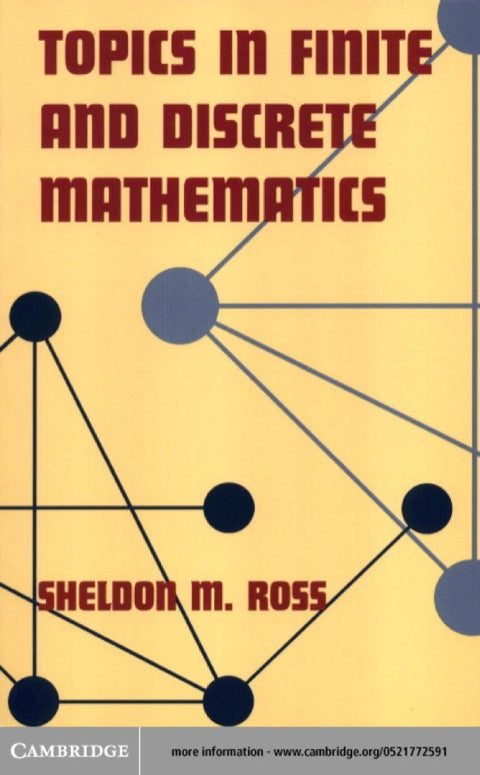# Topics in Finite and Discrete Mathematics

Sheldon M. Ross   ·  ISBN 9780511034541Zookal account needed
Get it instantly
\$62.70  Save \$3.11
\$59.59
 Publisher Cambridge University Press Author(s) Sheldon M. Ross Published 07312000 Related course codes
Written for a broad audience of students in mathematics, computer science, operations research, statistics, and engineering, this textbook presents a short, lively survey of several fascinating non-calculus topics in modern applied mathematics. Coverage includes probability, mathematical finance, graphs, linear programming, statistics, computer science algorithms, and groups. A key feature is the abundance of interesting examples not normally found in standard finite mathematics courses, such as options pricing and arbitrage, tournaments, and counting formulas. The author assumes a level of mathematical sophistication at the beginning calculus level, that is, students should have had at least a course in pre-calculus, and the added sophistication attained from studying calculus would be useful.